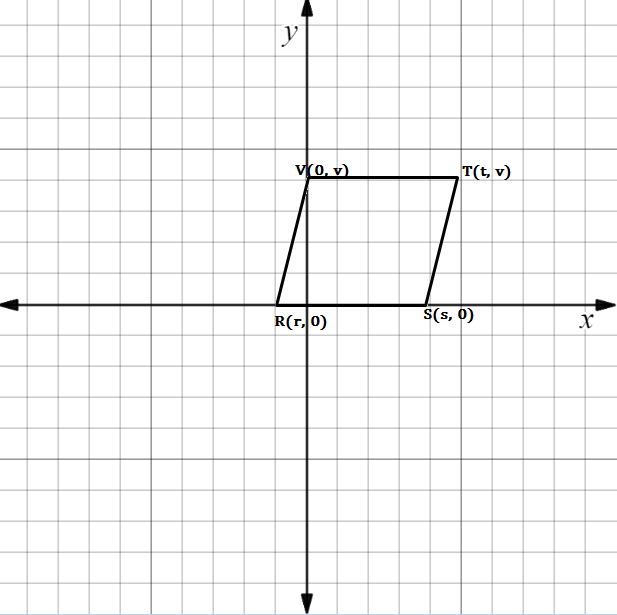Chapter 10.CT, Problem 15CTElementary Geometry For College St...

7th Edition
Alexander + 2 others
ISBN: 9781337614085

Solutions

Chapter
SectionElementary Geometry For College St...

7th Edition
Alexander + 2 others
ISBN: 9781337614085
Textbook Problem

In the figure, we see that m R S - = m V T - = 0 . Find the equation that relates r ,   s ,   a n d   t if it is known that R S T V is a parallelogram.To determine

To find:

The equation that relates r, s, and t if it is known that RSTV is a parallelogram.

Explanation

Given figure is the parallelogram RSTV.

Here the slope of RS=VT=0

That is mRS-=mVT-=0

And the slope of VR= the slope of TS

That is mVR-=mST-

Formula for slope of the line is m=y2-y1x2-x1

Slope of VR:

x1, y1=(0, v)

x2, y2=(r,0)

Substituting the above values in the formula,

mVR-=0-vr-0

mVR-=

Still sussing out bartleby?

Check out a sample textbook solution.

See a sample solution

The Solution to Your Study Problems

Bartleby provides explanations to thousands of textbook problems written by our experts, many with advanced degrees!

Get Started

In Exercises 1124, find the indicated limits, if they exist. 15. limx2x+3x29

Applied Calculus for the Managerial, Life, and Social Sciences: A Brief Approach

Evaluate the integrals in Problems 7-36. Check your results by differentiation. 34.

Mathematical Applications for the Management, Life, and Social Sciences

Evaluate the definite integral. 01xex2dx

Single Variable Calculus: Early Transcendentals, Volume I

The third partial sum of is:

Study Guide for Stewart's Single Variable Calculus: Early Transcendentals, 8th